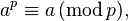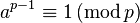# Fermat's little theorem facts for kids

Kids Encyclopedia Facts

Fermat's little theorem is a theorem from number theory. It is named after Pierre de Fermat who found it in the 17th century. It is about the properties of primes. It says that if a is a number, and p is a prime, then$a^p \equiv a\,(\mathrm{mod}\,p),$

If a is not a multiple of p, then the following is often used:$a^{p-1} \equiv 1\,(\mathrm{mod}\,p)$

## Images for kidsFermat's little theorem Facts for Kids. Kiddle Encyclopedia.